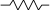# Raymii.orgQuis custodiet ipsos custodes?

## Only zero is false, everything else is true in C++

Published: 12-07-2019 | Author: Remy van Elst | Text only version of this article

Some of the code I work on does the following, mostly because it's older C style code now used in a C++ context:

• Have a value that can be changed by a user.
• If that value is not set, it is set to `-1`
• That variable, when set to a number, is what a function will use

Zero in this context means that it can be changed but is set to 0. `-1` sometimes means it can not be edited, but sometimes means it's off. Most of the cases I find where this is used do it this way to save memory.

(This gives a host of other problems, like, how to preserve the value when (turning it off and later just turning it back on?)

Sometimes this variable is checked for true-ness by using a boolean conversion, like this:

``````if (variable) {
do_stuff();
}
``````

Only if the variable is zero, this check will not execute. If it's set to `-1`, the boolean conversion will convert to `true`, which might not be what you meant. What you want to check for is:

``````if (variable > 0) {
do_stuff();
}
``````

But better would be to have a seperate variable for the `on/off` and a seperate variable for the actual value to use.

This is oversimplified and for seasoned programmers this will be nothing new, however I found it interesting.

### Implicit conversion rules to booleans

The rules for implicit conversion, which is what's happening when you use something else as a boolean, are described here.

Quoting:

A prvalue of integral, floating-point, unscoped enumeration, pointer, and pointer-to-member types can be converted to a prvalue of type bool. The value zero (for integral, floating-point, and unscoped enumeration) and the null pointer and the null pointer-to-member values become false. All other values become true.

Here is my example code:

``````#include <iostream>

int main () {
bool boolMinOne;
bool boolPlusOne;
bool boolZero;
bool boolnullptr;
bool boolPtr;

int intPlusOne { 1 };
int intMinOne { -1 };
int intZero { 0 };

int* intPtr { &intPlusOne };
int* nullPtr { nullptr };

boolMinOne = intMinOne;
boolPlusOne = intPlusOne;
boolZero = intZero;
boolPtr = intPtr;
boolnullptr = nullPtr;

std::cout << "boolMinOne: " << boolMinOne << "\n";
std::cout << "boolPlusOne: " << boolPlusOne << "\n";
std::cout << "boolZero: " << boolZero << "\n";
std::cout << "boolNullptr: " << boolnullptr << "\n";
std::cout << "boolPtr: " << boolPtr << "\n";

return 0;
}
``````

Result:

``````boolMinOne: 1
boolPlusOne: 1
boolZero: 0
boolNullptr: 0
boolPtr: 1
``````
Tags: c , c++ , conversion , cpp , development , snippets